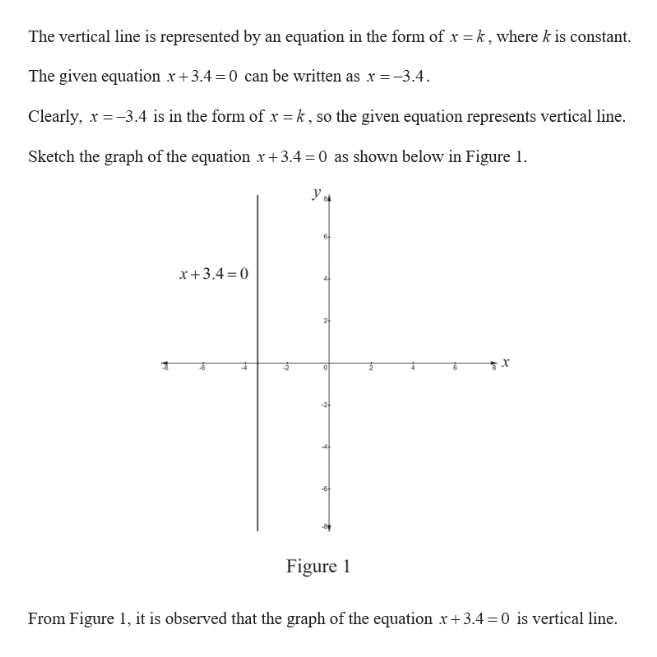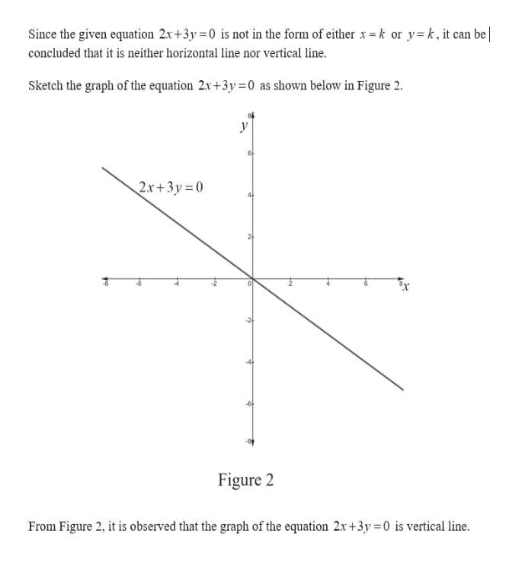Determine whether each equation could represent a vertical line, a horizontal line, or neither. a.     x+3.4=0  b. 2x+3y=0 c.     y-10=0

Question

Determine whether each equation could represent a vertical line, a horizontal line, or neither.

a.     x+3.4=0

b. 2x+3y=0

c.     y-10=0

Step 1

a. Determine whether x+3.4=0 represents a horizontal line or vertical line.help_outlineImage TranscriptioncloseThe vertical line is represented by an equation in the form of x k, wherek is constant. The given equation x+3.4-0 can be written as x -3.4. Clearly, 3.4 is in the form of xk, so the given equation represents vertical line. Sketch the graph of the equation r+3.4 0 as shown below in Figure 1 x+3.4 0 Figure 1 From Figure , it is observed that the graph of the equation x+3.4 0 is vertical line fullscreen
Step 2

b.2x+3y=0

...help_outlineImage TranscriptioncloseSince the given equation 2x+3y 0 is not in the form of either x= k or y concluded that it is neither horizontal line nor vertical line. k, it can be Sketch the graph of the equation 2x+3y-0 as shown below in Figure 2 2x+3y=0 Figure 2 From Figure 2, it is observed that the graph of the equation 2x+3y 0 is vertical line. fullscreen

Want to see the full answer?

See Solution

Want to see this answer and more?

Our solutions are written by experts, many with advanced degrees, and available 24/7

See Solution
Tagged in

Other